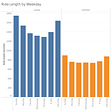# Cyclistic Case Study

````{r setup, include=FALSE}knitr::opts_chunk\$set(echo = TRUE)``````{r Environment Setup}library(tidyverse)library(readr)library(lubridate)library("here")library("skimr")library("janitor")nov20 <- read_csv("202011-divvy-tripdata.csv")dec20 <- read_csv("202012-divvy-tripdata.csv")jan21 <- read_csv("202101-divvy-tripdata.csv")feb21 <- read_csv("202102-divvy-tripdata.csv")mar21 <- read_csv("202103-divvy-tripdata.csv")apr21 <- read_csv("202104-divvy-tripdata.csv")may21 <- read_csv("202105-divvy-tripdata.csv")jun21 <- read_csv("202106-divvy-tripdata.csv")jul21 <- read_csv("202107-divvy-tripdata.csv")aug21 <- read_csv("202108-divvy-tripdata.csv")sep21 <- read_csv("202109-divvy-tripdata.csv")oct21 <- read_csv("202110-divvy-tripdata.csv")````

• A description of all data sources used
• Documentation of any cleaning or manipulation of data
• A summary of your analysis
• Supporting visualizations and key findings
• How do annual members and casual riders use Cyclistic bikes differently?
• Why casual riders would buy a membership?
• How digital media could affect their marketing tactics?

# Prepare and Process the Data

• Reliable: This data is reliable as there is unlikely to be sampling bias given that I am using the data wholesale from Cyclistic.
• Original: This data is considered original as it is downloaded directly from Cyclistic (first-party data).
• Comprehensive: The data is comprehensive given that it contains many details for each individual trip.
• Current: The data is current as it is the previous twelve months of data.
• Cited: The data is first-hand data.

## Structure

• A ride_id to identify each ride individually
• rideable_type which identifies the type of bike used. There are 3 options: electric_bike, docked_bike, and classic_bike
• start and end date and time: started_at, ended_ at
• The starting and ending station names and ID — start_nation_name, start_station_id, end_station_name, end_station_id
• Detailed geographical coordinates of the starting and ending stations: start_lat,start_lng, end_lat, end_lng
• A boolean value detailing if a ride was by a casual rider or a member

## The Process

````{r Process and Clean The Data}ttm <- rbind(nov20, dec20, jan21, feb21, mar21, apr21, may21, jun21, jul21, aug21,              sep21, oct21)rm(nov20, dec20, jan21, feb21, mar21, apr21, may21, jun21, jul21, aug21, sep21, oct21)ttm<-ttm %>% mutate(year=year(started_at),month=month(started_at), day=day(started_at))ttm <- mutate(ttm, ride_length=ended_at - started_at)ttm[['ride_length']] <- hms::hms(seconds_to_period(ttm[['ride_length']]))ttm <- mutate(ttm,day_of_week=weekdays(started_at))ttm <- ttm %>% filter(ride_length>"0")ttm <- mutate(ttm, month= month.abb[month(started_at)])ttm <- ttm %>% drop_na()member <- ttm %>% filter(member_casual == "member")casual <- ttm %>% filter(member_casual == "casual")````

## Analysis

````{r Stat Tibbles}member_stats <- member %>% summarize(mean = mean(ride_length), sd = sd(ride_length),                                      number = nrow(member), max = max(ride_length),                                      min=min(ride_length), member_casual ="member")casual_stats <- casual %>% summarize(mean = mean(ride_length), sd = sd(ride_length),                                      number = nrow(member),max = max(ride_length),                                      min=min(ride_length), member_casual = "casual")stats <- rbind(member_stats, casual_stats)rm(member_stats, casual_stats)stats[["mean"]] <- hms::hms(seconds_to_period(stats[['mean']]))stats[["max"]] <- hms::hms(seconds_to_period(stats[['max']]))stats[["min"]] <- hms::hms(seconds_to_period(stats[['min']]))``````{r Plot Average Ride Duration}ggplot(data=ttm, aes(x=member_casual,y=ride_length))+  geom_bar(stat="summary",fun="mean",fill="paleturquoise4")+  labs(x="Type of Customer", y="Ride Duration (mm:ss)",        title = "Average Ride Duration of the Different Types of Customers",       subtitle ="Data Between Nov 2020 - Oct 2021")+  annotate("text",x=1,y=1100,label="33:00")+  annotate("text",x=2,y=500,label="13:28")````
````{r Average Rides by Types }ggplot(data=ttm)+  geom_bar(mapping=aes(x=member_casual, fill=rideable_type))+  labs(x="Type of Customer", y="Number of Rides",        title = "Average Rides by the Different Types of Customers",        subtitle ="Data Between Nov 2020 - Oct 2021")+  annotate("text",x=1,y=1100000,label="1,221,380")+  annotate("text",x=1,y=570000,label="350,276")+  annotate("text",x=1,y=200000,label="458,951")+  annotate("text",x=2,y=1100000,label="1,836,978")+  annotate("text",x=2,y=570000,label="112,468")+  annotate("text",x=2,y=200000,label="511,270")````
````{r Month Plot}ttm\$month <-factor(ttm\$month,levels=c("Nov","Dec","Jan","Feb","Mar",                                      "Apr","May","Jun","Jul","Aug","Sep","Oct"))ggplot(ttm,aes(x=month,fill=member_casual))+  geom_bar()+  labs(x="Month", y="Number of Rides", title = "Total Number of Rides in Each Month",        subtitle ="Data Between Nov 2020 - Oct 2021")+  scale_y_continuous(labels= scales::comma)````
````{r Date Plot}ggplot(ttm,mapping=aes(x=day,fill=member_casual))+  geom_bar()+  labs(x="Day", y="Number of Rides", title = "Total Rides on each Date",        subtitle ="Data Between Nov 2020 - Oct 2021",       caption="Note that data for the 31st is lower than the        rest as some months do not have the 31st")+  scale_y_continuous(labels= scales::comma)````
````{r Week Date}ttm\$day_of_week <-factor(ttm\$day_of_week,levels=c("Sunday","Monday","Tuesday",                                                  "Wednesday","Thursday","Friday", "Saturday"))ggplot(ttm,aes(x=day_of_week,fill=member_casual))+  geom_bar()+  labs(x="Day", y="Number of Rides", title = "Weekly Distribution of Rides",        subtitle ="Data Between Nov 2020 - Oct 2021")+  scale_y_continuous(labels= scales::comma)````
````{r Station Counter}start_station_count <- ttm%>% select(start_station_name) %>%   count(start_station_name,sort =TRUE)start_station_count <- head(start_station_count,10)end_station_count <- ttm%>% select(end_station_name) %>%   count(end_station_name,sort =TRUE)end_station_count <- head(end_station_count,10)``````{r Station Plot}ggplot(data=start_station_count, aes(x=start_station_name, y =n))+  geom_col(fill="paleturquoise4")+  coord_flip()+  labs(x="Station Name", y= "Frequency", title = "Frequent Starting Stations",       subtitle = "Data Between Nov 2020 - Oct 2021")+  geom_text(aes(label=n),hjust=1.2)ggplot(data=end_station_count, aes(x=end_station_name, y =n))+  geom_col(fill="paleturquoise4")+  coord_flip()+  labs(x="Station Name", y= "Frequency", title = "Frequent Ending Stations",        subtitle = "Data Between Nov 2020 - Oct 2021")+  geom_text(aes(label=n),hjust=1.2)````

# Change Log

• Combined all to form a single Data Frame (ttm)
• Split started_at into year, month and date columns
• Created a new column called “ride_length” which is the difference between “start_at” and “ended_at”
• Convert ride_length to data type
• Created day_of_week using started_at
• Removed all negative ride_length. Rows decreased from 5,378,834 to 5,376,953
• Dropped all rows with NULL values. Rows decreased from 5,376,953 to 4,491,323
• Created casual tibble of dimensions 2,030,607 x 18
• Created member tibble of dimensions 2,460,716 x 18
• Created stats tibble of dimensions 2 x 6

--

--

--

## More from Lim Wei Hern

Singaporean. Interested in Economics, Finance and Data Analytics

Love podcasts or audiobooks? Learn on the go with our new app.

## These 5 Benefits of Spreadsheets Make It a Vital Skill## Recommendation Engine/ Next Best Product — Part 1 -The Basics## D4S Sunday Briefing #65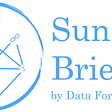## Stories without structure## From Groups to Individuals: Permutation Testing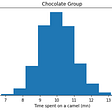## Cultivating Digital DNA Enables a Thriving Real-World Evidence Ecosystem to Improve Patient…## Technical ability as a data analist is overrated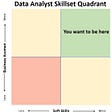## Lim Wei Hern

Singaporean. Interested in Economics, Finance and Data Analytics

## Bellabeat Case Study## 3 reasons why every Data Analyst should be a Data Storyteller.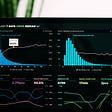## Cyclistic Case Study